## POLAR

 Applies either a rectangular (cartesian) to polar transformation or a polar to rectangular (cartesian) transformation on an image.

 USAGE: polar [-m mode] [-c xc,yc] [-r rmn,rmx] [-a amn,amx] [-v vpmode] [-b bgcolor] infile outfile USAGE: polar [-h or -help] -m .... mode ......... rect2polar or polar2rect; default=rect2polar -c .... xc,yc ........ coordinates of the transformation center; ...................... default is image center -r .... rmn,rmx ...... min and max radius; default=0,(half image diagonal); ...................... non-negative floats; rmx can be w, h, d or m for ...................... width/2, height/2, diagonal/2 or min(width,height)/2 of image -a .... amn,amx ...... min and max angle; default=-180,180; ...................... floats between -180 and 180 -v .... vpmode ....... virtual-pixel mode to use to fill area of output image ...................... that are outside the input image; default=edge -b .... bgcolor ...... background color for virtual-pixel=background ...................... any valid IM color is allowed. The default=black PURPOSE: To apply either a rectangular (Cartesian) to polar transformation or a polar to rectangular (Cartesian) transformation on an image. DESCRIPTION: POLAR applies either a rectangular (Cartesian) to polar transformation or a polar to rectangular (Cartesian) transformation on an image. The user has control of the range of radius, angle and center in the transformation. ARGUMENTS: -m mode ... MODE is the transformation mode, which can be either rect2polar (rectangular to polar) or polar2rect (polar to rectangular). The former, rect2polar, treats the input as a normal rectangular image with columns representing X and rows representing Y and treats the output as having columns representing angle and rows representing radius. The latter, polar2rect, treats the input as having columns representing angle and rows representing radius and treats the output as having columns representing X and rows representing Y. Note that in a polar image, nominally the left column is angle=amn=-180, the middle column is angle=0 and the right column is angle=amx=180. Similarly, the top row is radius=rmn=0 and the bottom row is radius=rmx=(half image diagonal). -c xc,yc ... XC,YC are the pixel coordinates for the transformation center. The default is the image center. -r rmn,rmx ... RMN,RMX are the min and max radii. By default, rmn=0 and rmx=(half image diagonal). This provides a transformation that includes the whole image. However, for the rect2polar mode, this will cause the output image to contain areas that are outside the input image. They will be filled as determined by the virtual pixel setting. Value are non-negative floats. Values are generally non-negative floats, but four other choices are allowed: w, h, d and m, where w=width/2, h=height/2, d=diagonal/2 and m=min(width,height)/2. -a amn,amx ... AMN,AMX are the min and max angles. By default, amn=-180 and amx=180. Values for amn and amx are floats between -180 and 180. -v vpmode ... VPMODE is the virtual-pixel mode. Any valid IM virtual-pixel setting is allowed. The default is edge. -b bgcolor ... BGCOLOR is the background color to use with vpmode=background. Any valid IM color may be used. The default is black. Note that this script may be slow due to the use of -fx. CAVEAT: No guarantee that this script will work on all platforms, nor that trapping of inconsistent parameters is complete and foolproof. Use At Your Own Risk.

### EXAMPLES

 Rectangular To Polar Transformation -- Full 360 Degree Range, Max Radius = Diagonal/2, Vary Y Center Coordinate Original Image (red dot inserted at center) Rect2Polar Arguments: -m rect2polar -c 49.5,49.5 -r 0,h -a -180,180 -v black Rect2Polar Arguments: -m rect2polar -c 49.5,30 -r 0,h -a -180,180 -v black Rect2Polar Arguments: -m rect2polar -c 49.5,10 -r 0,h -a -180,180 -v black Rect2Polar Arguments: -m rect2polar -c 49.5,0 -r 0,h -a -180,180 -v black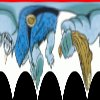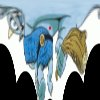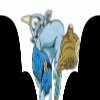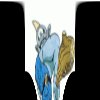Rectangular To Polar Transformation -- 180 Degree Range, Max Radius = Diagonal/2, Vary Center Y Coordinate Original Image (red dot inserted at center) Rect2Polar Arguments: -m rect2polar -c 49.5,49.5 -r 0,h -a -90,90 -v black Rect2Polar Arguments: -m rect2polar -c 49.5,30 -r 0,h -a -90,90 -v black Rect2Polar Arguments: -m rect2polar -c 49.5,10 -r 0,h -a -90,90 -v black Rect2Polar Arguments: -m rect2polar -c 49.5,0 -r 0,h -a -90,90 -v black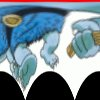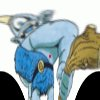Rectangular To Polar Transformation Followed By Polar To Rectangular Original Image (red dot inserted at center) Rect2Polar (rmax=diagonal/2) (-180<=angle<=180) Arguments: -m rect2polar -r 0,h -v white Polar2Rect On Rect2Polar Image Arguments: -m polar2rect -r 0,h -v white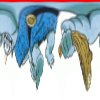Original Image (red dot inserted at center) Rect2Polar (rmax=min(width,height)/2) (-180<=angle<=180) Arguments: -m rect2polar -r 0,m -v black Polar2Rect On Rect2Polar Image Arguments: -m polar2rect -r 0,m -v black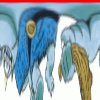Original Image (red dot inserted at center) Rect2Polar (rmax=diagonal/2) (-90<=angle<=90) (y center = 0) Arguments: -m rect2polar -c 49.5,0 -r 0,m -a -90,90 -v black Polar2Rect On Rect2Polar Image Arguments: -m polar2rect -c 49.5,0 -r 0,h -a -90,90 -v black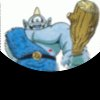Polar To Rectangular Transformation -- Full 360 Degree Range Original Image (red dot inserted at center) Polar2Rect (rmax=diagonal/2) Arguments: -m polar2rect -r 0,h -v black Polar2Rect (rmax=min(width,height)/2) Arguments: -m polar2rect -r 0,m -v white Polar2Rect (rmax=diagonal/2) (original image flipped) Arguments: convert -flip -m polar2rect -r 0,h -v black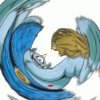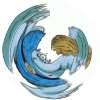Polar To Rectangular Transformation -- Vary Angular Range, Max Radius = 100, Center Y = 0 Original Image (red dot inserted at center) Polar2Rect Arguments: -m polar2rect -c 49.5,0 -r 0,100 -a -90,90 -v black Polar2Rect Arguments: -m polar2rect -c 49.5,0 -r 0,100 -a -60,60 -v black Polar2Rect Arguments: -m rect2polar -c 49.5,0 -r 0,100 -a -30,30 -v black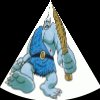Polar To Rectangular Transformation -- Full 360 Degree Range, Center Y = 0, Variation In Radii Original Image (red dot inserted at center) Polar2Rect (rmin=0) (rmax=diagonal/2) Arguments: -m polar2rect -c 49.5,0 -r 0,h -v black Polar2Rect (rmin=20) (rmax=diagonal/2) Arguments: -m polar2rect -c 49.5,0 -r 20,h -v black Polar2Rect (rmin=20) (rmax=100) Arguments: -m polar2rect -c 49.5,0 -r 20,100-v black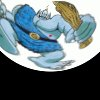Polar To Rectangular Followed By Rectangular To Polar Transformation Original Image (red dot inserted at center) Polar2Rect (rmax=max(width,height)/2) (-180<=angle<=180) Arguments: -m rect2polar -r 0,m -v white Rect2Polar On Polar2Rect Image Arguments: -m polar2rect -r 0,m -v whiteReal World Application -- Convert 360 Degree "Fisheye" View To Strip Panorama And Extract Subsection Example Follows Procedure Outlined At www.0-360.com Note that this picture is not from a true fisheye lens. It is from a 360 degree panoramic lens with 115 degree vertical field of view. But the way the image is recorded makes it look like a fisheye image. Original ImageTrimmed To Size Of Circle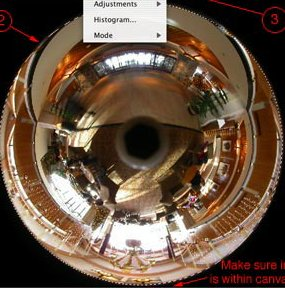Polar Transform Arguments -mode rect2polar -r 0,mFlip Image convert -flipResize Image 100% x 39% convert -resize 100%x39%!Crop Image As Desired convert -crop ...Enlarge convert -resize 400%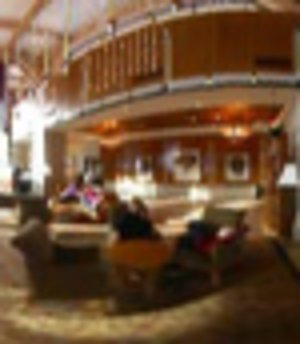Radial Blur specify blur in pixels in radial direction polar -m rect2polar convert blur to percentages to scale down and to resize back up to do the blurring scale down in vertical direction, then resize up vertically to original size polar -m polar2rect infile="flower.jpg" blur=20 inname=`convert \$infile -format "%t" info:` polar -m rect2polar \$infile \${inname}_rect2polar.jpg frac=`convert \$infile -format "%[fx:1/\$blur]" info:` min=`convert xc: -format "%[fx:100*\$frac]" info:` max=`convert xc: -format "%[fx:100/\$frac]" info:` convert \${inname}_rect2polar.png -scale 100x\${min}% -resize 100x\${max}% \${inname}_rect2polar_radial\${blur}.jpg polar -m polar2rect \${inname}_rect2polar_radial\${blur}.jpg \$inname_radial\${blur}.jpg Original ImageImage In Polar Coordinates Arguments: -m rect2polar20 Pixel Vertically Blurred Polar Image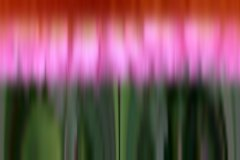Image Back In Cartesian (Rectangular) Coordinates Arguments: -m polar2rectAngular Blur specify blur in degrees in angular direction polar -m rect2polar convert blur to percentages to scale down and to resize back up to do the blurring scale down in horizontal direction, then resize up horizontally to original size polar -m polar2rect infile="flower.jpg" blur=10 inname=`convert \$infile -format "%t" info:` polar -m rect2polar \$infile \${inname}_rect2polar.jpg frac=`convert \$infile -format "%[fx:360/(\$blur*w)]" info:` min=`convert xc: -format "%[fx:100*\$frac]" info:` max=`convert xc: -format "%[fx:100/\$frac]" info:` convert \${inname}_rect2polar.png -scale \${min}x100% -resize \${max}x100% \${inname}_rect2polar_angular\${blur}.jpg polar -m polar2rect \${inname}_rect2polar_angular\${blur}.jpg \$inname_angular\${blur}.jpg Original ImageImage In Polar Coordinates Arguments: -m rect2polar10 Pixel Horizontally Blurred Polar ImageImage Back In Cartesian (Rectangular) Coordinates Arguments: -m polar2rect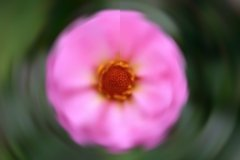Image Rotation polar -m rect2polar roll the image -- the roll amount as a fraction of width will be the rotation amount polar -m polar2rect infile="cyclops1.jpg" rotation=180 inname=`convert \$infile -format "%t" info:` polar -m rect2polar \$infile \${inname}_rect2polar.jpg rollamt=`convert \$infile -format "%[fx:w*\$rotation/360]" info:` convert \${inname}_rect2polar.jpg -roll +\${rollamt}+0 \${inname}_rect2polar_roll\${rollamt}.jpg polar -m polar2rect \${inname}_rect2polar_roll\${rollamt}.jpg \${inname}_rect2polar_roll\${rollamt}_polar2rect.jpg Original ImageImage In Polar Coordinates Arguments: -m rect2polar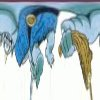Roll Image To Rotate 180 deg -roll +50+0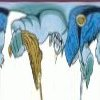Image Back In Cartesian (Rectangular) Coordinates Arguments: -m polar2rect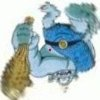What the script does is as follows: Applies an rectangular to polar or polar to rectangular warp to an image Uses -fx; therefore slow This is equivalent to the following IM commands for the case of a rectangular to polar warp: compute image dimensions for default center and max radius values convert \$infile -virtual-pixel edge -monitor \ -fx "yy=((j-\$rmn)*\$rmx/h)*cos(((\$amx-\$amn)*pi/180)*(i/(w-1))+(\$amn*pi/180))+\$yc; \ xx=((j-\$rmn)*\$rmx/h)*sin(((\$amx-\$amn)*pi/180)*(i/(w-1))+(\$amn*pi/180))+\$xc; u.p{xx,yy}" \ \$outfile And this is equivalent to the following IM commands for the case of a polar to rectangular warp: compute image dimensions for default center and max radius values convert \$infile -virtual-pixel edge -monitor \ -fx "rr=hypot((i-\$xc),(j-\$yc)); aa=atan2((i-\$xc),(j-\$yc)); \ xx=(aa-(pi/180)*\$amn)*(w-1)/((pi/180)*(\$amx-\$amn)); \ yy=(rr-\$rmn)*(h-1)/(\$rmx-\$rmn); u.p{xx,yy}" \ \$outfile